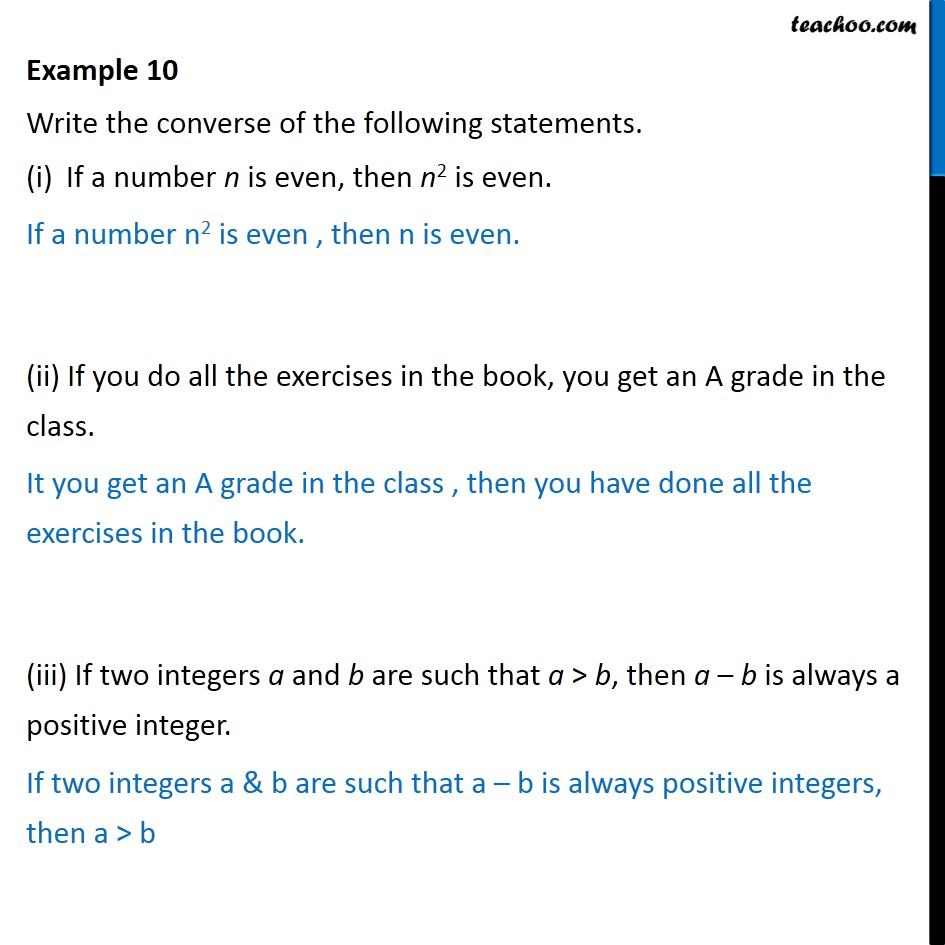1. Chapter 14 Class 11 Mathematical Reasoning
2. Serial order wise
3. Examples

Transcript

Example 10 Write the converse of the following statements. If a number n is even, then n2 is even. If a number n2 is even , then n is even. (ii) If you do all the exercises in the book, you get an A grade in the class. It you get an A grade in the class , then you have done all the exercises in the book. (iii) If two integers a and b are such that a > b, then a – b is always a positive integer. If two integers a & b are such that a – b is always positive integers, then a > b

Examples